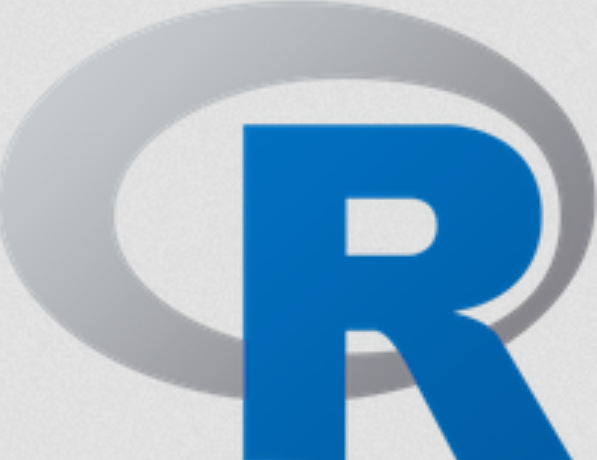# K Means Clustering in RK Means Clustering is an unsupervised learning algorithm that tries to cluster data based on their similarity.

Unsupervised learning means that there is no outcome to be predicted, and the algorithm just tries to find patterns in the data. In k means clustering, we have the specify the number of clusters we want the data to be grouped into. The algorithm randomly assigns each observation to a cluster, and finds the centroid of each cluster.

Then, the algorithm iterates through two steps:

• Reassign data points to the cluster whose centroid is closest
• Calculate new centroid of each cluster

These two steps are repeated till the within-cluster variation cannot be reduced any further. The within-cluster variation is calculated as the sum of the euclidean distance between the data points and their respective cluster centroids.

Exploring the data
The iris dataset contains data about sepal length, sepal width, petal length, and petal width of flowers of different species. Let us see what it looks like: Continue reading## Share:

Subscribe
Notify ofInline Feedbacks

On social media